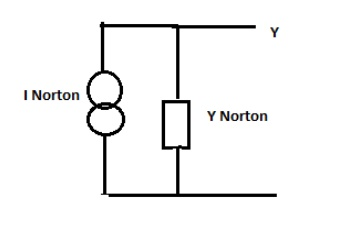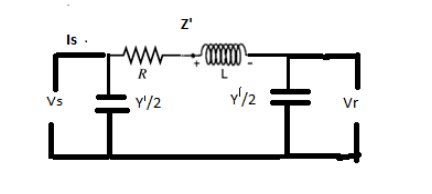Home | | Power System Analysis | Modeling of Components For Load Flow Analysis

# Modeling of Components For Load Flow Analysis

The thevenins equivalent circuit of the generator i.e. The voltage source in series with the thevenins equivalent impedance. Z = R + jX

MODELING OF COMPONENTS FOR LOAD FLOW ANALYSIS

## Generator models Generators:

The thevenins equivalent circuit of the generator i.e. The voltage source in series with the thevenins equivalent impedance. Z = R + jXThe Norton form equivalent circuit of the generator i.e. The current source in parallel with the admittance## Transformer model## Transmission system model

Transmission Line

Transmission line are modelled as

(i). Short line model

(ii). Medium line model

(iii). Long line model

(i). Short line model : Resistance & inductance are assumed to be lumped### Medium line model (lines between 80 to 250km)

Resistance &inductance are assumed to be lumped &the total shunt admittance is divided in to two equal parts & placed at the receiving and sending ends.X = Lω

Y/2 = Cω/2

A = 1+ZY/2

B=Z

C=Y(1+ZY/4)

D=1+ZY/4

### Long line model (lines above 250)Z’=Z sinhγL / γ L

Y’/2 = 1/Zc tan h (γL/2)### Shunt Elements:

The shunt capacitor is connected to bus i. If S is MVAR rating of shunt capacitor. So is base MVA admittance P.u. Y P.u. = 0+jS/S0Shunt reactors is connected io bus i. If S is MVAR rating of shunt capacitor. So is base MVA admittance P.u. Y P.u. = 0-jS/S0